5. Canonical formalism.

Consider the action integral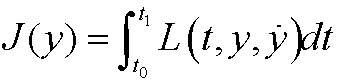and the corresponding Lagrange equationWe define a new variable p, called the canonical momentum, byIf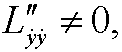we might according to the implicit function theorem solve foras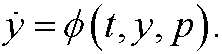In particular we may write the Hamiltonian asExample 4: Consider a particle of mass m and potential energy V(y), moving in one dimension. Then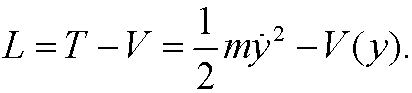This implies that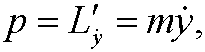the regular momentum. If we solve forwe getThe Hamiltonian thus becomes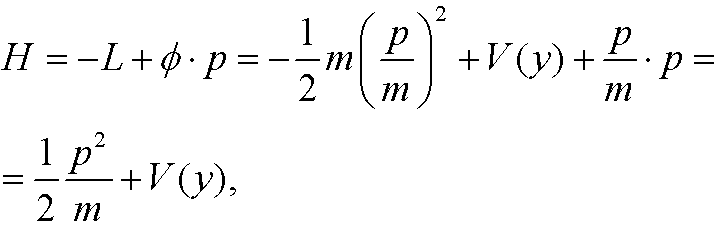the total energi expressed in the position y and the momentum p.

We also note that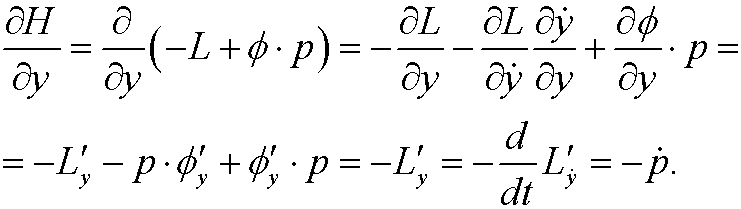Lagrange’s equations can thus be written as a system of equations in the variables y and p,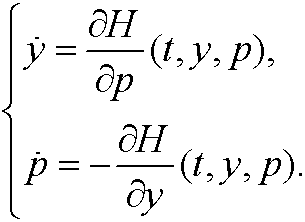Definition: The equations above are called Hamilton’s equations.

Example 5: Consider the harmonic oscillator in Example 1. ThenHamilton’s equations are in this caseWe solve these equations in the yp-plane (the so called  phase plane). Division yieldsThis is a separable equation. Integration giveswhere C is a constant. Geometrically this is a family of ellipses in the phase plane.This are the curves the system develops along in the phase space.
Remark: Euler-Lagrange’s equation for the harmonic oscillator iswith solutions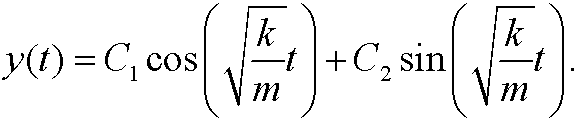These solutions can also be represented in the yt-plane, shown below.